output.to from Sideway
Draft for Information Only

# Forces

The effect of force on an object can be characterized by its point of application, magnitude, and direction. As force has both magnitude and direction, it is a vector quantity. The unit of force is Newton.

Force Vectors

A force vector can be represented by an arrow with the length of arrow represents the magnitude of the force, the angle between the arrow and the coordinate axis defines the direction of force indicated by the line of action, and the arrow head indicates the sense of direction.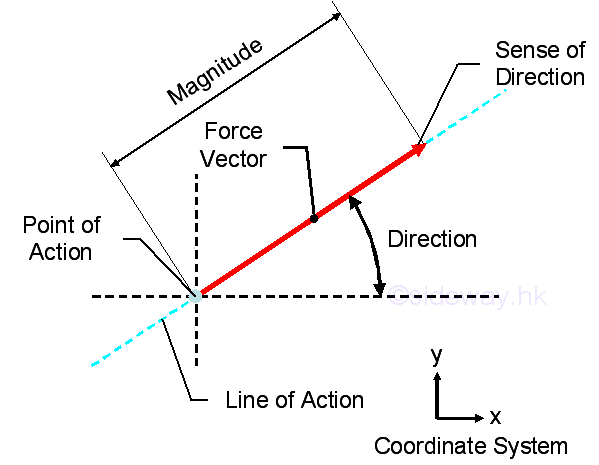Forces in a Plane

When more than one force act on a point, they can be replaced by a single resultant force with teh same effect on the point of action.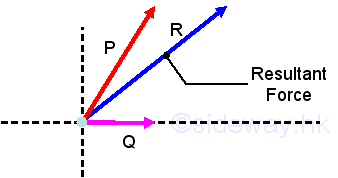The resultant force can be determined by means of vector addition.

• Parallelogram Law: The applied forces can be represented by the adjacent sides of the parallelogram and the resultant force can be obtained by drawing the diagonal of the parallelogram.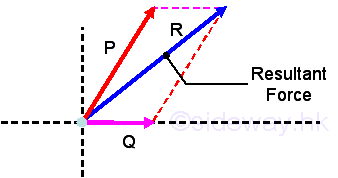• Triangle Rule: The applied force can be represented by two sides of a triangle in sequence and the resultant force can be obtained by drawing the third closing side of the triangle.in the opposite sense. Similarly, the triangle rule can further extend to the polygon rule, by using polygon construction method to represent the resultant force in both magnitude and direction.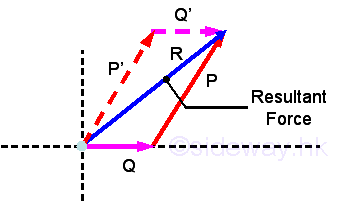• Laws of Trigonometric Functions : This is an analytical method based on the geometry instead of using vector construction method with true scale of magnitude and direction.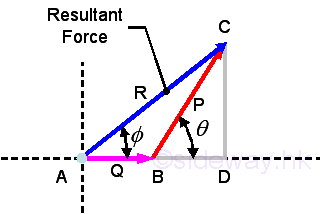The magnitude of the resultant froce can be determined by pythagorean theorem or the law of cosines.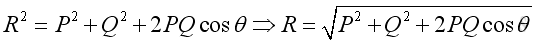The direction of the resultant froce can be determined by the law of sines.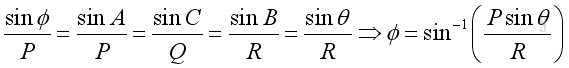or be determined by the triangle rules.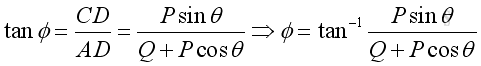Force decomposition

From the parallelogram Law, force can be resolved into two components. When the two components are perpendicular to each other in the form of a rectangle, they are called rectangular components.or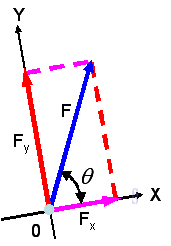Assumed two unit vectors, i and j, with unit magnitude along the x and y axis. Then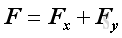,, and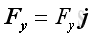, imply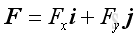The two components of the force vector can be obtained by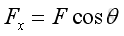andThe magnitude of the force vector can also be obtained by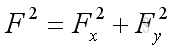And the direction of the force vector can also be obtained by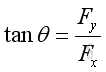Force Equilibrium

According to Newton's first law of motion, when a particle is in equilibrium, the resultant of all the forces acting on it is zero. That isAnd graphically in the form of force polygon,, implyWhen there are only three applied forces, the problem can be reduced to a force triangle and be solved by trigonometry.

Analytically, forces can be resolved into rectangular components to form the equations of equilibrium. Imply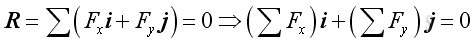Therefore,and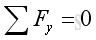ID: 110400006 Last Updated: 30/4/2011 Revision: 0 Ref:References

1. I.C. Jong; B.G. rogers, 1991
2. F.P. Beer; E.R. Johnston,Jr.; E.R. Eisenberg, 2004Home 5

Management

HBR 3

Information

Recreation

Culture

Chinese 1097

English 337

Computer

Hardware 149

Software

Application 187

Numeric 19

Programming

Web 757

CSS 1

HTML

Knowledge Base

OS 389

MS Windows

Knowledge

Mathematics

Algebra 20

Geometry 18

Calculus 67

Engineering

Mechanical

Rigid Bodies

Statics 92

Dynamics 37

Control

Physics

Electric 10